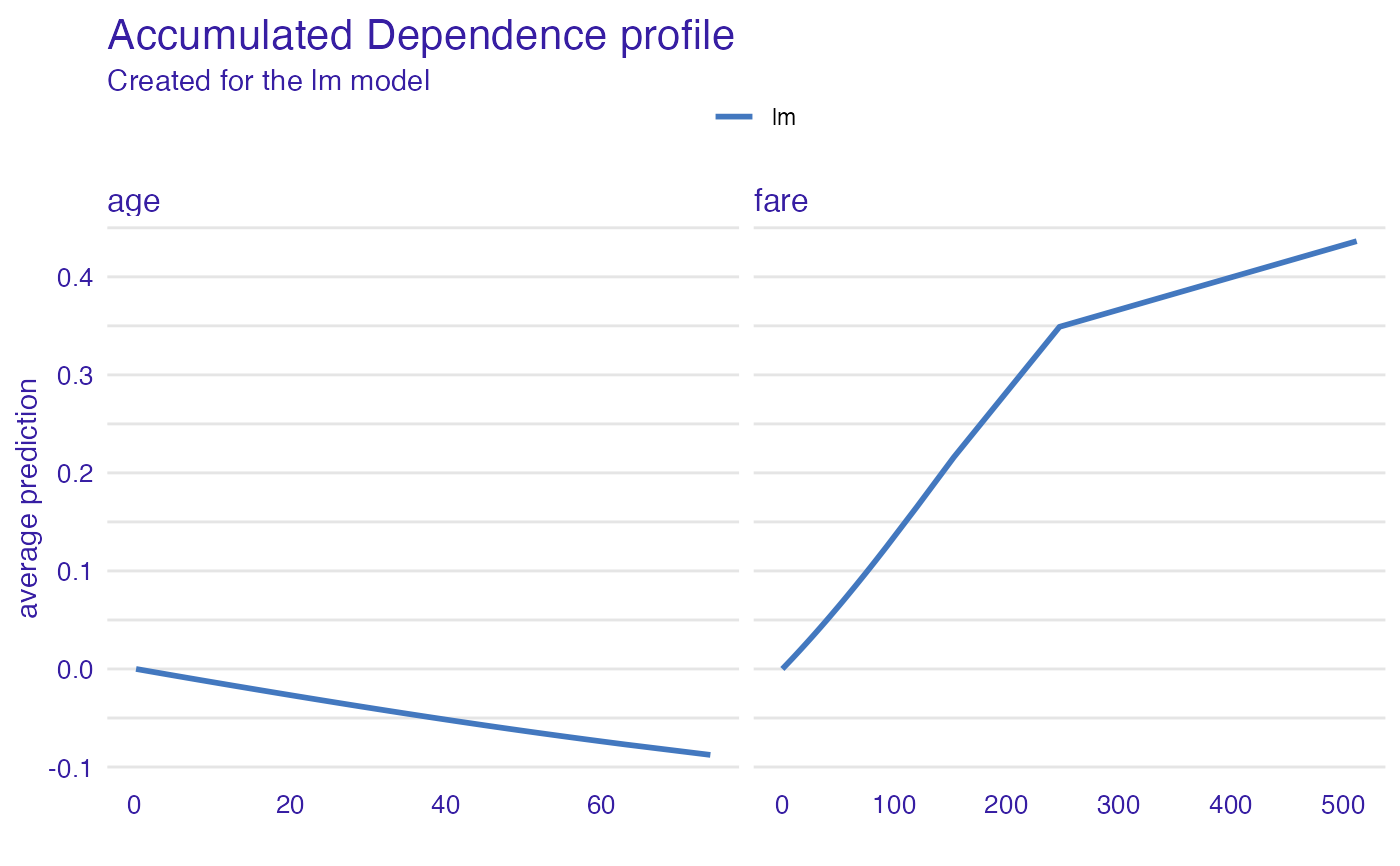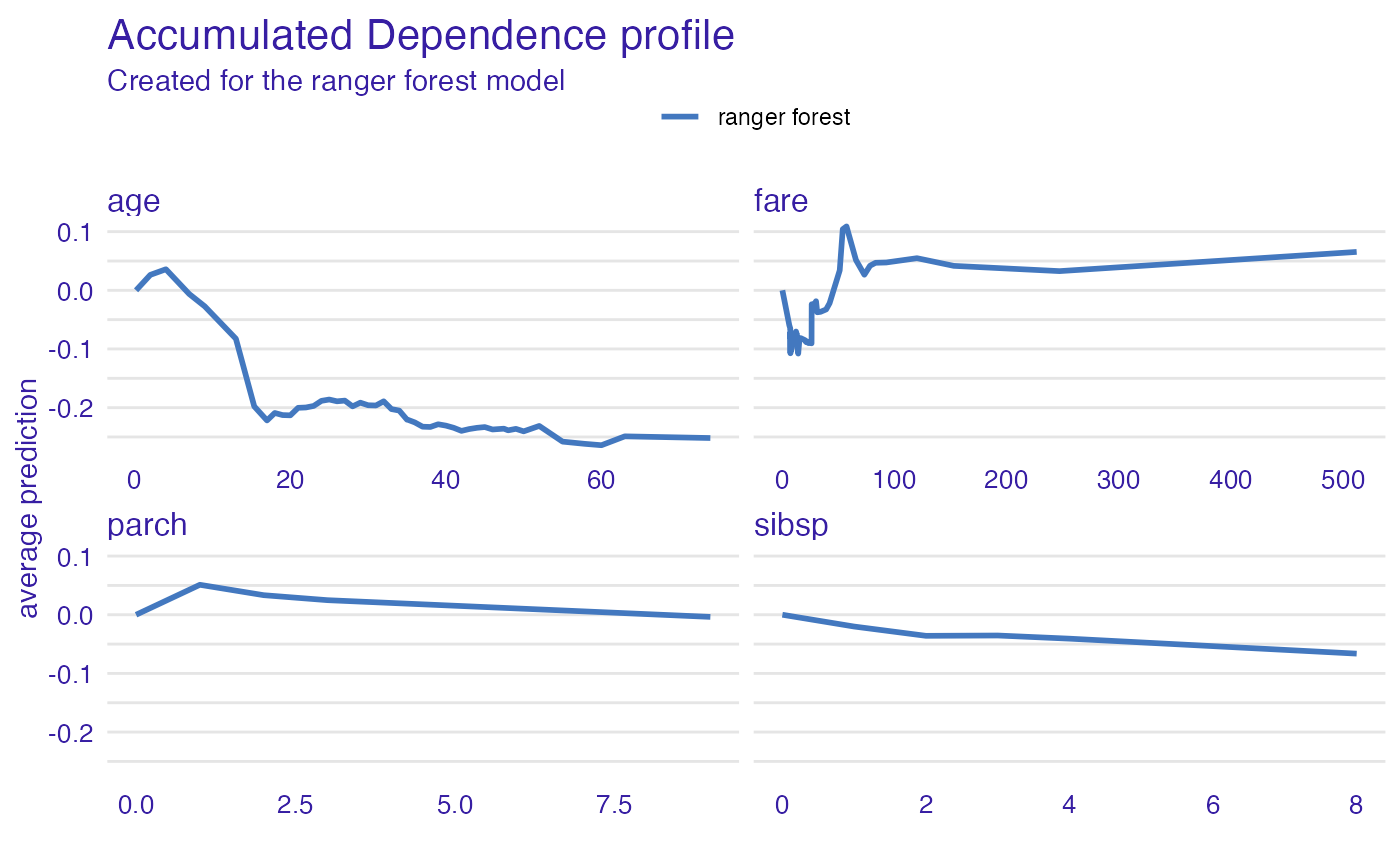Accumulated Local Effects Profiles accumulate local changes in Ceteris Paribus Profiles. Function accumulated_dependence calls ceteris_paribus and then aggregate_profiles.

accumulated_dependence(x, ...)

# S3 method for explainer
accumulated_dependence(
x,
variables = NULL,
N = 500,
variable_splits = NULL,
grid_points = 101,
...,
variable_type = "numerical"
)

# S3 method for default
accumulated_dependence(
x,
data,
predict_function = predict,
label = class(x),
variables = NULL,
N = 500,
variable_splits = NULL,
grid_points = 101,
...,
variable_type = "numerical"
)

# S3 method for ceteris_paribus_explainer
accumulated_dependence(x, ..., variables = NULL)

accumulated_dependency(x, ...)

## Arguments

x an explainer created with function DALEX::explain(), an object of the class ceteris_paribus_explainer or a model to be explained. other parameters names of variables for which profiles shall be calculated. Will be passed to calculate_variable_split. If NULL then all variables from the validation data will be used. number of observations used for calculation of partial dependence profiles. By default, 500 observations will be chosen randomly. named list of splits for variables, in most cases created with calculate_variable_split. If NULL then it will be calculated based on validation data avaliable in the explainer. number of points for profile. Will be passed tocalculate_variable_split. a character. If "numerical" then only numerical variables will be calculated. If "categorical" then only categorical variables will be calculated. validation dataset Will be extracted from x if it's an explainer NOTE: It is best when target variable is not present in the data predict function Will be extracted from x if it's an explainer name of the model. By default it's extracted from the class attribute of the model

## Value

an object of the class aggregated_profiles_explainer

## Details

Find more detailes in the Accumulated Local Dependence Chapter.

ALEPlot: Accumulated Local Effects (ALE) Plots and Partial Dependence (PD) Plots https://cran.r-project.org/package=ALEPlot, Explanatory Model Analysis. Explore, Explain, and Examine Predictive Models. https://ema.drwhy.ai/

## Examples

library("DALEX")
#> Welcome to DALEX (version: 2.3.0).
#> Find examples and detailed introduction at: http://ema.drwhy.ai/
#> Additional features will be available after installation of: ggpubr.
#> Use 'install_dependencies()' to get all suggested dependencies#>
#> Attaching package: ‘DALEX’#> The following object is masked from ‘package:ingredients’:
#>
#>     feature_importancelibrary("ingredients")

model_titanic_glm <- glm(survived ~ gender + age + fare,
data = titanic_imputed, family = "binomial")

explain_titanic_glm <- explain(model_titanic_glm,
data = titanic_imputed[,-8],
y = titanic_imputed[,8],
verbose = FALSE)

N = 25, variables = c("age", "fare"))
#> Top profiles    :
#>   _vname_ _label_        _x_       _yhat_ _ids_
#> 1     age      lm  0.1666667  0.000000000     0
#> 2     age      lm  2.0000000 -0.002551359     0
#> 3     age      lm  4.0000000 -0.005323191     0
#> 4     age      lm  7.0000000 -0.009455409     0
#> 5     age      lm  9.0000000 -0.012193262     0
#> 6     age      lm 13.0000000 -0.017620564     0plot(adp_glm)# \donttest{
library("ranger")

model_titanic_rf <- ranger(survived ~., data = titanic_imputed, probability = TRUE)

explain_titanic_rf <- explain(model_titanic_rf,
data = titanic_imputed[,-8],
y = titanic_imputed[,8],
label = "ranger forest",
verbose = FALSE)

adp_rf <- accumulated_dependence(explain_titanic_rf, N = 200, variable_type = "numerical")